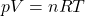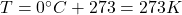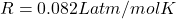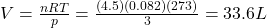## What is the volume of 4.5 mol of an ideal gas at a pressure of 3 atm and a temperature of 0° C? 1 liter = 0.001 m and 1 atm = 10

Question

What is the volume of 4.5 mol of an ideal gas
at a pressure of 3 atm and a temperature of
0° C? 1 liter = 0.001 m and 1 atm = 101300
Pascals.

in progress 0
2 months 2021-08-03T16:37:57+00:00 1 Answers 0 views 0

33.6 L

Explanation:

We can solve the problem by using the equation of state for ideal gases, which states that:where

p is the pressure of the gas

V is its volume

n is the number of moles

R is the gas constant

T is the absolute temperature of the gas

For the ideal gas in this problem we have:

n = 4.5 mol (number of moles)

p = 3 atm (pressure)is the temperatureis the gas constant

Solving for V, we find the volume of the gas: